# Operation of Matrices## Operation of Matrices

Here in Operation of Matrices, we deal with the Addition of Matrices, Subtraction of Matrices and Multiplication of a Matrix by a scalar (real number).

********************

10 Math Problems officially announces the release of Quick Math Solver, an Android App on the Google Play Store for students around the world.

********************

If A and B are two matrices of the same order, then A and B are said to be Conformable or Compatible for addition. The sum of A and B is denoted by A + B and it is obtained by adding corresponding elements of matrices A and B.The matrix A + B will be of the same order as each of the matrices A and B is.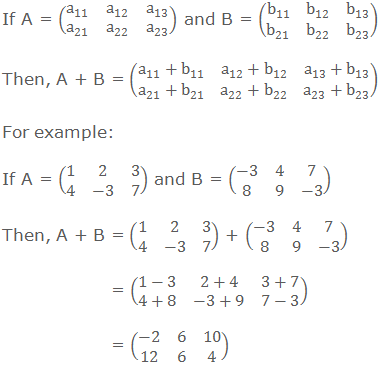### Subtraction of Matrices

If A and B are two matrices of the same order, then they are said to be conformable for subtraction. The difference of the matrix B from A is denoted by A – B and it is obtained by subtracting the elements of B from the corresponding elements of A.

The order of the matrix A – B is same as the order of A or B.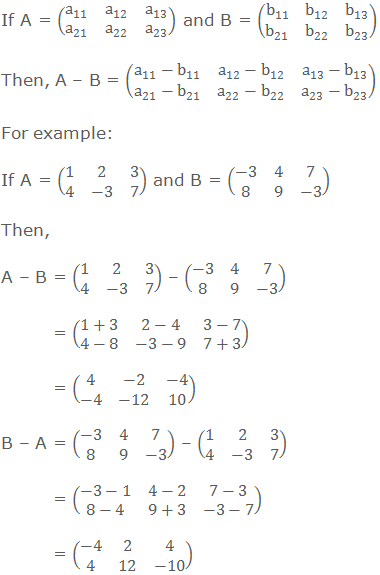### Multiplication of a Matrix by a scalar (real number)

If A is any matrix and k is any constant or a scalar, then the matrix obtained by multiplying each element of A by k is denoted by kA and it is called scalar multiple of A by k.

The order of the matrix kA is the same as the order of the matrix A.### Algebraic Properties of Matrix Addition

The addition of matrices satisfies the following properties:

1.   Closure property:

If A and B are two matrices of the same order, then their sum A + B is also a matrix of the same order as that of A or B.2.   Commutative property:

If A and B are two matrices of the same order, then A + B = B + A.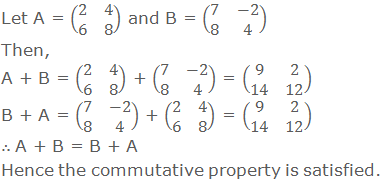3.   Associative properties:

If A, B and C are three matrices of the same order, then (A + B) + C = A + (B + C)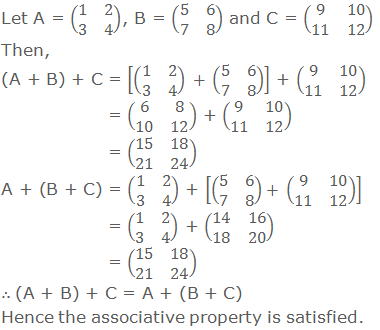If A is any matrix, then there exists a null matrix O of the same order such as A + O = O + A = A.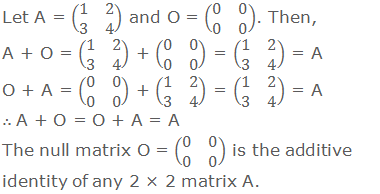If A is a matrix of any order, then there exists another matrix –A or same order such that A + (-A) = (-A) + A = O, the additive identity.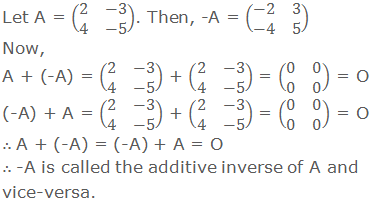6.   If A and B are the matrices of the same order and k is a scalar, then k(A + B) = kA + kB.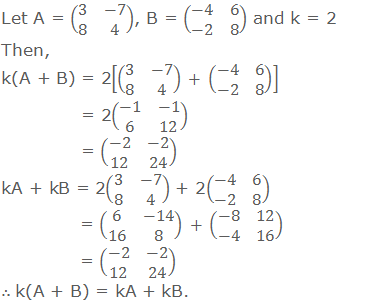7.   If A is a matrix and c, k are any two scalars, then (c + k)A = cA + kA.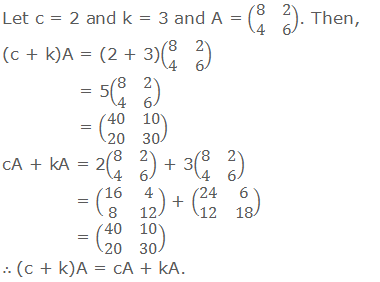8.   If c, k are any two scalars and A is a matrix, then c(kA) = (ck)A.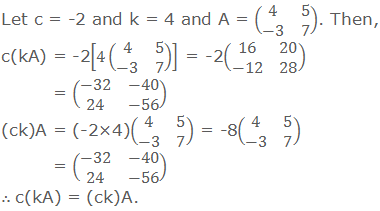Worked Out Examples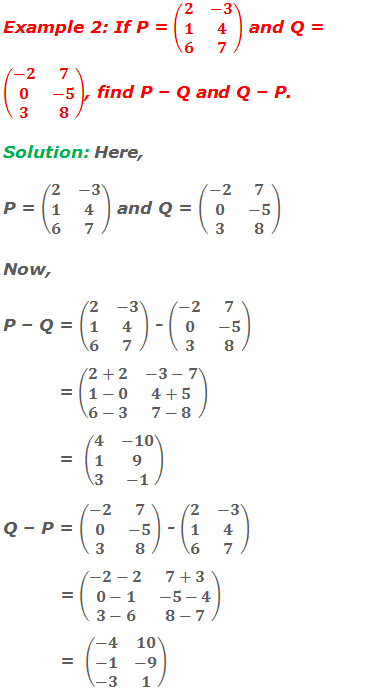#### Do you have any questions regarding the enlargement and reduction?

You can ask your questions or problems here, in the comment section below.